Home

 << Previous    1    3  4  5  ...24    Next >>

2. The 10×24 division in sacred geometries & its realisation in the 421 polytope representing the 240 roots of E8

Article 53 explains how the structural elements (yods or points, lines & triangles) of 2-dimensional and 3-dimensional sacred geometries divide in a non-trivial way into 10 sets of 24 elements. The table below summarises this 10-fold pattern.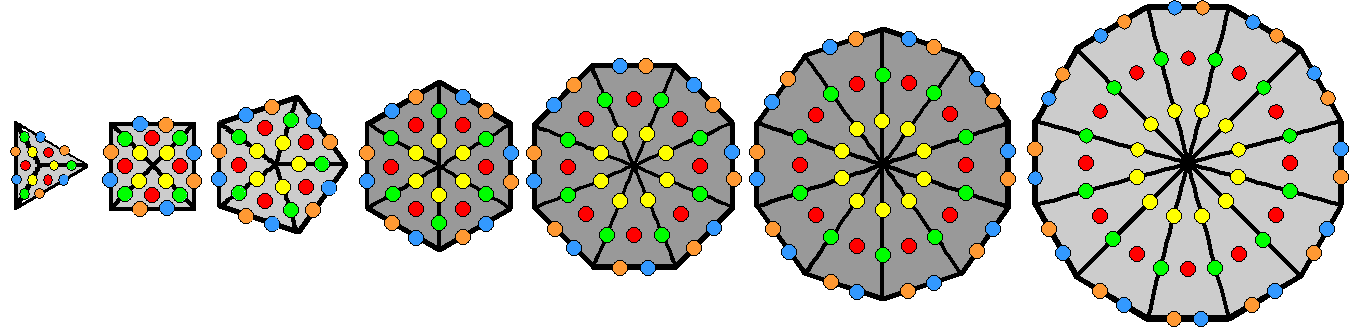The 4 light grey and 3 dark grey Type A regular polygons of the inner Tree of Life have (120+120=240) hexagonal yods.

The 7 polygons with 3, 4, 5, 6, 8, 10 & 12 corners can form 2 subsets, each with 24 corners, in 2 different ways:

(3+4+5+12) + (6+8+10)
(3+5+6+10) + (4+8+12).

However, given the analogy between the 7 polygons and the 7 Sephiroth of Construction which, in order of emanation, form two triads and a singlet, only the first way is consistent with this 3:3:1 pattern because the second way has one of the polygons (square) in the first triplet of polygons (coloured light grey) being grouped with the octagon in the second triplet S of polygons (dark grey), which violates this pattern. The triangle, square & pentagon have 12 sectors with (12×5=60) hexagonal yods, as does the dodecagon. The set of these 4 polygons will be called "S." The hexagon, octagon & decagon in S have 24 sectors with (24×5=120) hexagonal yods. The 7 polygons contain 240 hexagonal yods made up of 120 hexagonal yods in S and 120 hexagonal yods in S′. They are distributed 5 per sector, coloured red, orange, yellow, green & blue. Each colour is repeated 24 times in S (write the set as 24) and 24 times in S′ (write it as 24). Hence, the 240 hexagonal yods naturally group into 5 sets of 24 and 5 sets
of
24, i.e., 10 sets of 24 hexagonal yods:

240 = 120 + 120,

where

120 = 5×24 and 120′ = 5×24′.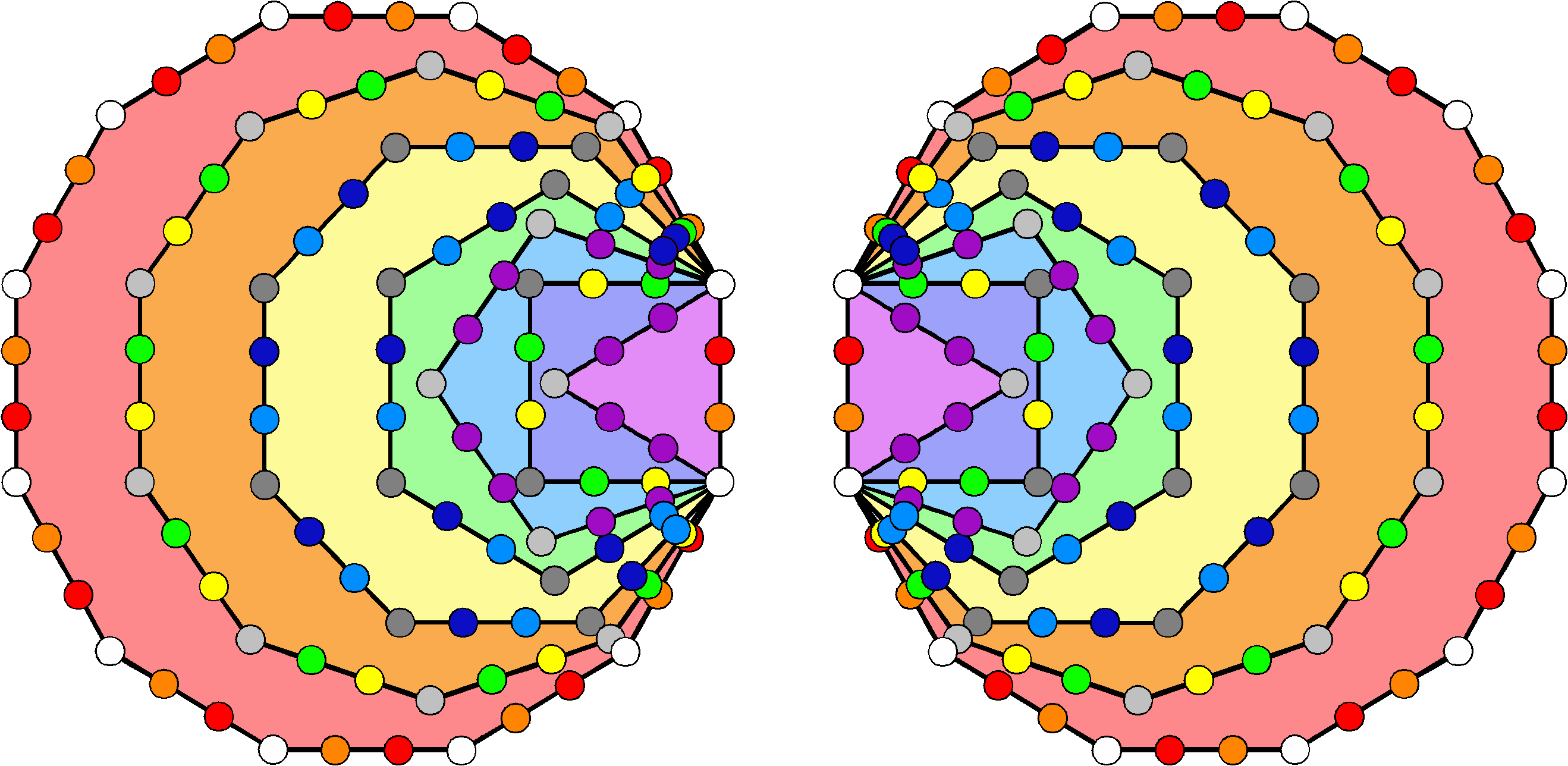120 yods as 10 sets of 12 line the sides of each separate set of seven enfolded Type A polygons.

Taken separately, the two halves of the inner form of the Tree of Life consist of 7 regular polygons enfolded in one another so that they have 36 corners.  These form 3 sets of 12:

1. dodecagon: 12 white corners;
2. triangle, pentagon & decagon: 12 light grey corners;
3. square, hexagon & octagon: 12 dark grey corners.

The 84 hexagonal yods on the sides of each set of 7 enfolded polygons form 7 sets of 12:

1. The 2 sides of the triangle outside the root edge have 4 violet hexagonal yods. The 4 external sides of the pentagon have 8 violet hexagonal yods. Hence, the 2 polygons have 12 violet hexagonal yods;
2. The 5 external sides of the hexagon have 5 indigo hexagonal yods and the 7 external sides of the octagon have 7 indigo hexagonal yods. Hence, these 2 polygons have 12 indigo hexagonal yods;
3. The 5 external sides of the hexagon and the 7 external sides of the octagon have 12 blue hexagonal yods;
4. The 3 external sides of the square and the 9 external sides of the decagon have 12 green hexagonal yods;
5. The 3 external sides of the square and the 9 external sides of the decagon have 12 yellow hexagonal yods;
6. The 12 sides of the dodecagon have 12 orange hexagonal yods;
7. The 12 sides of the dodecagon have 12 red hexagonal yods.

The 120 yods on the boundary of each set of enfolded polygons form 10 sets of 12 yods. Both sets of enfolded polygons form 10 sets of 24 yods.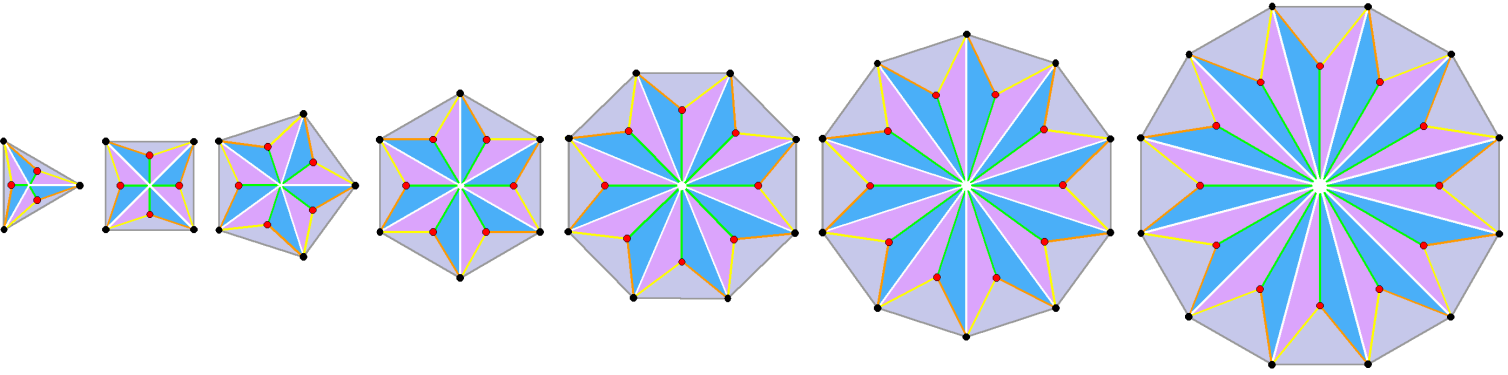10 classes of 24 geometrical elements surround the centres of the triangle, square,
pentagon & dodecagon, as well as the centres of the hexagon, octagon & decagon.

Each sector of a Type B n-gon is a Type A triangle. It has 10 geometrical elements per sector surrounding the centre of the n-gon:

1. black, polygonal corner;
2. grey polygonal side;
3. white, radial side;
4. red corner shared by 3 triangles;
5. orange side of triangle;
6. yellow side of triangle;
7. green side of triangle;
8. blue triangle;
9. indigo triangle;
10. violet triangle.

Surrounding the centres of the Type B triangle, square & pentagon are 120 geometrical elements that form 10 classes of 12. Surrounding the centre of the Type B dodecagon are 120 geometrical elements in 10 classes of 12 elements. The centres of the 4 polygons in S are surrounded by 240 geometrical elements that form 10 classes of 24, as are the centres of the 3 polygons in S′. The 10 classes of geometrical elements comprise 5 points & triangles and 5 lines. Hence, the 240 geometrical elements in S or S′ consist of 5 sets of 24 points & triangles and 5 sets of lines, i.e., 10 sets of 24 geometrical elements.Surrounding the centre of the Type B dodecagon are 120 geometrical elements. They consist of 5 sets of 12 points & triangles and 5 sets of 12 lines, i.e., 5 sets of 24 geometrical elements. The 240 geometrical elements that surround the centres of 2 separate Type B dodecagons consist of 10 sets of 24 geometrical elements, i.e., 5 sets of 24 points & triangles and 5 sets of 24 lines.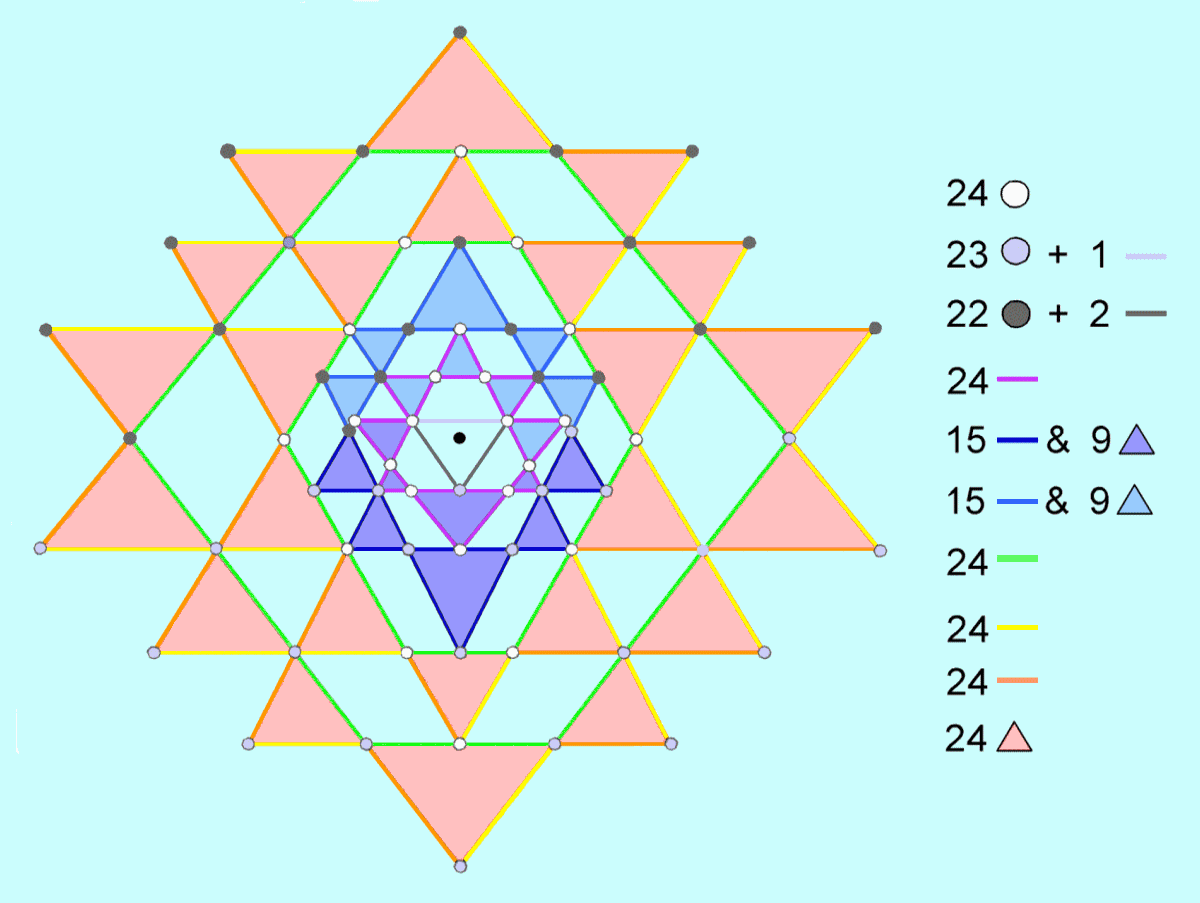Surrounding the centre of the 2-d Sri Yantra are 10 sets of 24 geometrical elements.

In the 2-dimensional Sri Yantra, the most well-known yantra in Hindu Tantra, 9 triangles (5 downward-pointing triangles, four upward-pointing triangles) are interlaced in such a way as to generate 43 smaller triangles. Arranged around a central triangle are 42 triangles. They form 4 groups: 8, 10, 10 & 14 triangles. At the centre of the central triangle is a point, or bindu, from which all levels of existence emanate. Because this point lies in the plane of the central triangle and is a distinct point, the latter is not a true triangle like the 42 other triangles in the true mathematical sense of being an area filled with points and bounded by 3 straight lines. It is, simply, a triangular boundary composed of 3 points and 3 straight lines. The table below lists the number of corners (points), sides of triangles (lines) & triangles in the 2-d Sri Yantra.

 Number of corners Number of sides Number of triangles Total Central 'triangle' 1 3 0 4 1st group of triangles 4 + 8 = 12 8×3 = 24 8 44 2nd group of triangles 6 + 10 = 16 10×3 = 30 10 56 3rd group of triangles 2 + 10 = 12 10×3 = 30 10 52 4th group of triangles 28 14×3 = 42 14 84 Total 69 129 42 240

For purposes of counting corners, the two upper corners of the central 'triangle,' which coincide with the points of contact of 2 pairs of triangles, are included with the first group of triangles. Including the lowest corner of the central 'triangle,' 69 points surround the centre of the 2-d Sri Yantra, as well as 129 lines & 42 triangles. The 240 geometrical elements surrounding the centre comprise 3 lines belonging to the central triangle and 69 points, i.e., 72 points & lines, and 168 lines & triangles. The former divide into 3 sets of 24 and the latter divide into 7 sets of 24:

1. 1st & 3rd groups have 24 white corners;
2. Central 'triangle', 2nd & 4th groups comprise 22 light grey corners in one half of Sri Yantra, lower light grey corner & upper light grey side of central 'triangle';
3. Central 'triangle', 2nd & 4th groups comprise 22 dark grey corners in other half & 2 dark grey sides of central 'triangle';
4. 3rd & 4th groups comprise 24 red triangles;
5. 3rd & 4th groups comprise 24 orange sides;
6. 3rd & 4th groups comprise 24 yellow sides;
7. 3rd & 4th groups comprise 24 green sides;
8. 1st & 2nd groups comprise in one half of Sri Yantra 15 blue sides & 9 blue triangles, i.e., 24 blue sides & triangles;
9. 1st & 2nd groups comprise in other half 15 indigo sides & 9 indigo triangles, i.e., 24 indigo sides & triangles;
10. 1st group comprises 24 violet sides.

The 240 points, lines & triangles comprise 10 sets of 24. The lack of mirror symmetry about the horizontal axis passing through the bindu of the distribution of 42 triangles around the central one means that not every set of 24 geometrical elements belonging to them can divide about this axis into two sets of 12. However, the diagram is symmetric about the vertical axis passing through the bindu and the 9 tips of the downward-pointing and upward-pointing triangles in the central column. Division by this central, vertical axis into two sets of 120 geometrical elements — one set the mirror image of the other — is meaningful provided that the 13 points, lines & triangles in the half of the Sri Yantra above the bindu that are aligned with this axis be formally associated with one half (left or right) of the diagram and their 13 counterparts in the half below the bindu be associated with the other half (right or left) of the diagram. Notice that YAHWEH with number value 26 prescribes the number of geometrical elements spanning this vertical axis.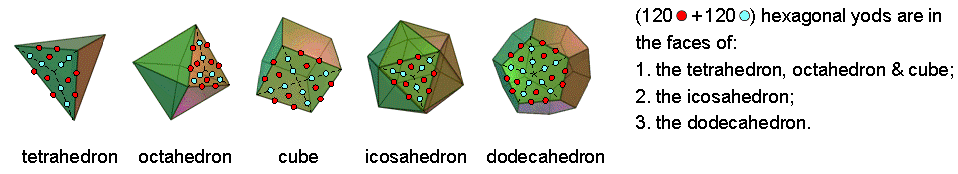The faces of the first three Platonic solids, the icosahedron & the dodecahedron have 10 sets of 24 hexagonal yods.

The hexagonal yod populations in the faces of the 5 Platonic solids.

 Platonic solid Edges Faces Hexagonal yods at centres Hexagonal yods on edges Hexagonal yods on interior sides Total Tetrahedron 6 4 4×3 = 12 6×2 = 12 4×6 = 24 48 Octahedron 12 8 8×3 = 24 12×2 = 24 8×6 = 48 = 2×24 96 Cube 12 6 6×4 = 24 12×2 = 24 6×8 = 48 = 2×24 96 Subtotal 30 18 60 60 120 240 Icosahedron 30 20 20×3 = 60 30×2 = 60 20×6 = 120 240 Subtotal 60 38 120 120 240 480 Dodecahedron 30 12 12×5 = 60 30×2 = 60 12×10 = 120 240 Total 90 50 180 180 360 720

When their 50 faces are constructed from tetractyses, the 5 Platonic solids have (720=6!) hexagonal yods. They are of 3 types: 1. hexagonal yods at centres of tetractyses; 2. hexagonal yods on edges of polyhedra, and 3. hexagonal yods on sides of tetractyses inside the polygonal faces. The first 3 Platonic solids have 240 hexagonal yods comprising 120 red hexagonal yods either at centres of tetractyses or on edges and 120 turquoise hexagonal yods on interior sides, as indicated in the pink row in the table. The former comprises (12+12=24) hexagonal yods at the centres of the faces of the tetrahedron or on its edges and 4 sets of 24 hexagonal yods either at centres or on edges, i.e., 5 sets of 24 hexagonal yods. The tetrahedron has 24 hexagonal yods on interior sides, whilst the octahedron has 48 such hexagonal yods (24 in each half), as does the cube. So the first 3 Platonic solids have 5 sets of 24 hexagonal yods on the interior sides of tetractyses in their faces. Altogether, they have 10 sets of 24 hexagonal yods that are made up of 2 groups of 5 sets of 24 yods (see the table shown above):

240 = 120 + 120 = [(12+12) + 24 + 24 + 24 + 24] + [24 + 2×24 + 2×24].

This 120:120 division in the 240 hexagonal yods in the faces of the first 3 Platonic solids re-occurs in both the icosahedron and the dodecahedron because they both have 120 hexagonal yods of the first two types and 120 hexagonal yods of the third type. Alternatively, each half of either polyhedron has (30+30+60=120) hexagonal yods (see the blue & red rows in the table).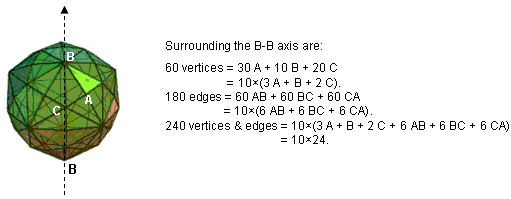10 sets of 24 vertices & edges surround an axis of the disdyakis triacontahedron.

The so-called "Catalan solids" are the duals of the more well-known Archimedean solids. The disdyakis triacontahedron is the Catalan solid with the most faces. It has 62 vertices. They are of three types: 30 A vertices are peaks of pyramids with Golden Rhombi as their bases. 12 B corners of these pyramids are vertices of an icosahedron with 20 triangular faces and their remaining 20 C corners are vertices of a dodecahedron with 12 pentagonal faces. If one imagines a straight line passing through two diametrically opposite B vertices of the disdyakis triacontahedron, then 30 A vertices, 10 B vertices & 20 C vertices surround this axis. 3 A vertices surround the C vertex at the centre of each icosahedral face with B vertices at its corners. This means that the 30 A vertices can be grouped into 10 sets of 3 belonging to the 10 pairs of opposite faces. The 60 vertices surrounding the B-B axis form 10 sets of 3 A vertices, 10 sets of one B vertex and a pair of diametrically opposite C vertices, i.e.,

30 A + 10 B + 20 C = 10×(3 A + B + 2 C).

The polyhedron has 180 edges (60 AB edges, 60 BC edges & 60 AC edges). The 20 equilateral triangles with B vertices at its corners are the faces of the underlying icosahedron, so that 3 AC edges are associated with each such triangle. Each icosahedral face has its diametrically opposite one. This means that there are 10 pairs of these triangles, i.e., 10 sets of 6 AC edges. A B vertex is the centre of a pentagonal face of the underlying dodecahedron. 5 AB edges meet at these centres, of which there are 6 pairs of diametrically opposite ones. The 60 AB edges therefore group into 10 sets of 6. Similarly, 5 BC edges meet at these centres, so that the 60 BC edges group into 10 sets of 6. The 180 edges group into 10 sets of (6 AB + 6 BC + 6 CA), i.e.,

60 AB + 60 BC + 60 CA = 10×(6 AB + 6 BC + 6 CA).

The (60+180=240) vertices & edges surrounding the B-B axis group into 10 sets of 24 vertices & edges:

30 A + 10 B + 20 C + 60 AB + 60 BC + 60 CA = 10×(3 A + B + 2 C + 6 AB + 6 BC + 6 CA).

Of course, this property is true whatever the axis is. But only in the case of a B-B axis are there 10 sets with identical geometrical compositions. Surrounding the axis in each half of the disdyakis triacontahedron are 120 vertices & edges. They are not grouped further into 10 identical sets of 12, because the odd numbers of A and B vertices in each set of 24 means that they cannot be divided equally into sets of 12. Instead, each half of the polyhedron is made up of 5 identical sets of 24 (=4×3×2×1) geometrical elements. The equation 120 = 5! = 5×4×3×2×1 = 5×4×6 is geometrically realised in the disdyakis triacontahedron because each of these 5 sets consists of 6 vertices and 3 sets of 6 edges, i.e., 4 sets of 6 vertices or edges.The 1-tree contains 10 sets of 24 yods that are not corners of its 19 Type A triangles.

The 1-tree contains 19 triangles with 11 corners and 50 sides. When they are Type A triangles, each with 10 internal yods, 3 corners & 2 hexagonal yods on every side, the number of yods in the 1-tree = 11 + 25×2 + 19×10 = 251. It contains 240 yods other than corners representing Sephiroth. The "trunk" of the 1-tree is the geometrical sequence:

1. point (Kether);
2. straight line (Chokmah-Binah Path);
3. triangle (corners at Chesed, Geburah & Tiphareth);
4. tetrahedron (vertices at Netzach, Hod, Yesod & Malkuth).

symbolised by the four rows of dots in the Pythagorean tetractys. Excluding corners representing Sephiroth, its yod population is tabulated below:

The yod population of the trunk of the Tree of Life.

 Corners Hexagonal yods at centres Hexagonal yods on sides of triangles Total Point 0 0 0 0 Line 0 0 2 2 Triangle 1 3 12 4 + 12 Tetrahedron 4 12 12 + 24 = 36 4 + (12+12=24) + 24 Total 5 15 50 (2+4+4=10) + 12 + 24 + 24= 70

The "root" of the 1-tree is its Path connecting Daath and Tiphareth, its projection onto the plane of the (7+7) enfolded polygons making up the inner form of the 1-tree being their root edge. Metaphysically, it is the interface between the outer form (trunk & branches) and inner aspects of the Tree of Life encoding the former. As the endpoints of this Path are Sephiroth, the number of non-Sephirothic yods in the root & trunk of the 1-tree = 2 + 70 = 72, where

72 = (10+2=12) + 12 + 24 + 24 = (12+12=24) + 24 + 24.

The 72 yods comprise 3 sets of 24 yods. This leaves 168 yods in the branches made up of 14 corners and 154 hexagonal yods in 14 Type A triangles with 2 hexagonal yods on each of their 14 sides (there are 15 sides but one of these is the root). There are 9 hexagonal yods inside each Type A triangle, so that the 168 yods comprise 14 corners, 14 sets of 9 hexagonal yods and 14 pairs of hexagonal yods. i.e., 14 sets of 12 yods, or 7 sets of 24 black yods. These sets are the 7 pairs of triangles making up the branches of the 1-tree, shown opposite as a dark & light prismatic colour. 5 pairs of mirror-image triangles numbered 3-7 are on opposite sides of the central Pillar of Equilibrium, which is straddled by 4 triangles as 2 pairs. One pair can be associated with one half of the 1-tree and the other pair can be associated with the other half (hence, they are depicted as light and dark shades of indigo and violet). The 240 extra yods needed to construct the 1-tree from Type A triangles comprise 10 sets of 24 yods — 3 sets in its root & trunk and 7 sets in its branches. For more details, see Article 53.

Given these remarkable correspondences between sacred geometries of various religions (as well as in polyhedra both well and little studied), it cannot be doubted that the exceptional Lie group E8 is central to the description of nature, for its number of roots is embodied in them all and it is improbable in the extreme to dismiss this as coincidence! The analysis presented below will reinforce this conclusion beyond all reasonable doubt.

The question raised by the embodiment in these sacred geometries of the number 240 and its factorisation into 10 sets of 24 is this: do the 240 roots of E8 form 10 groups of 24 as well? If so, this would create a striking parallel with the UPA, whose 10 whorls, each making 10 half-revolutions around its axis, were meticulously described by C.W. Leadbeater in the book Occult Chemistry that he co-authored with Annie Besant and for which this website presents evidence that it is the subquark state of the E8×E8 heterotic superstring. Indeed, it might amount to more than this. It might actually be hard proof that the UPA is such a superstring, thereby disproving — through past research by the author (see #12 in Occult Chemistry) showing that the UPA is the constituent of quarks — the assumption made by most particle physicists that quarks are superstrings.

The 421 polytope
Firstly, we need to consider a few geometrical concepts. A skew polygon is a polygon whose vertices do not lie in a plane. Skew polygons must have at least four vertices. A regular skew polygon has equal edge lengths. A facet of a polyhedron is any polygon whose corners are vertices of the polyhedron; it need not be a face. More generally, a facet of an n-polytope is an (n–1)-polytope formed by some of its vertices. n-dimensional facets are also called n-faces. For example, a 0-dimensional face is a vertex of a polyhedron, a 1-face is a 1-dimensional edge and a 2-face is a 2-dimensional face of a polyhedron. In higher-dimensional geometry, the facets of a n-polytope are the (n-1)-faces of dimension one less than the polytope itself. A Petrie polygon for a regular polytope of n dimensions is a skew polygon such that every (n1) consecutive sides (but no n) belong to one of the facets. For every regular polytope, there exists an orthogonal projection onto a plane such that one Petrie polygon becomes a regular polygon with the remainder of the projection interior to it. The plane in question is the Coxeter plane of the symmetry group of the polygon. Figure 1 shows the Petrie polygons for the five Platonic solids.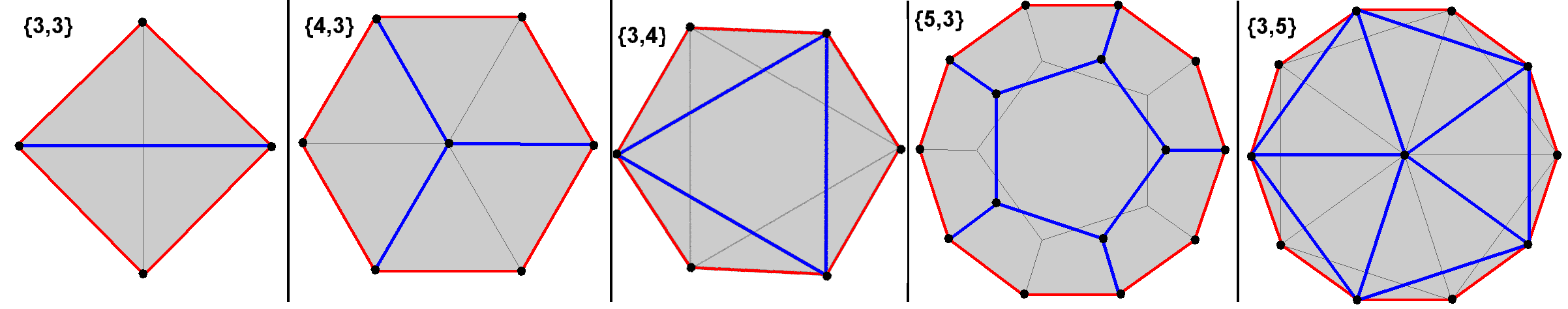(Blue lines show "front" edges, black lines show "back" edges.)

Figure 1. Petrie polygons for the Platonic solids (red edges). Depicted here is their orthogonal projection as regular planar polygons.
(uploaded by Tom Ruen to Wikipedia at http://commons.wikimedia.org/wiki/File:Petrie_polygons.png?uselang=en-gb ).

Figure 2 shows the Petrie polygons of the six polychorons and the 421 polytope to be discussed shortly.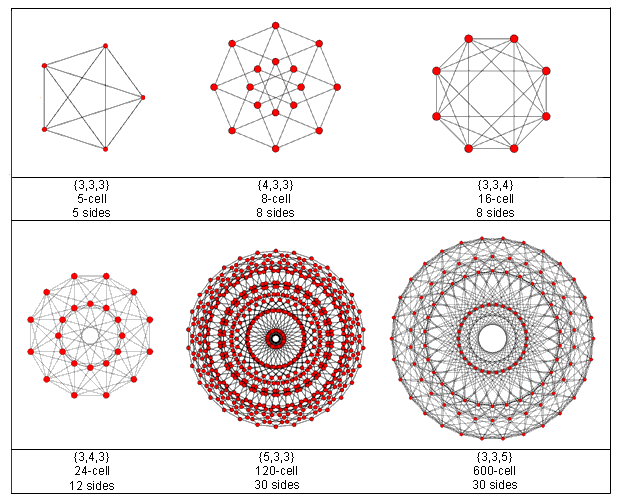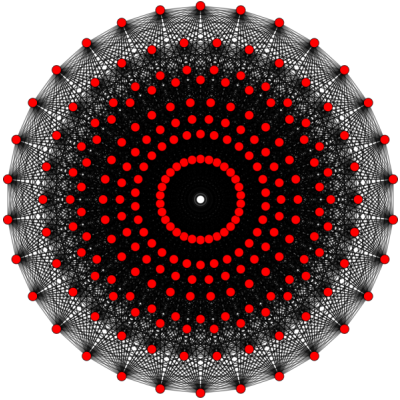The 6 polychorons. The 421 polytope. (Credit: " 2 41 t0 E8" by self - Own work. Licensed under Public domain via Wikimedia Commons).

Figure 2. The Petrie polygons of the six polychorons and the 421 polytope.

It is amusing that the pentagram, which the ancient followers of Pythagoras displayed on their arms as a public sign of their membership in his brotherhood, should appear in the Petrie polygon of the 5-cell, which is the simplest, 4-dimensional, regular polyhedron. They would certainly have approved, given their emphasis of the central role of the number 4 in the mathematics that describes nature, as enshrined in the author’s Tetrad Principle formulated in Article 1 and as demonstrated in nearly every one of his previous articles! The Petrie polygon of this polyhedron is a simple pentagon because the 5‑cell is dual to itself.

In 1900, Thorold Gosset (1869-1962), an English lawyer and amateur mathematician, discovered an 8‑dimensional, semi-regular polytope that has proved to be relevant to the theory of superstrings (or at least to the mathematics of one of the two symmetry groups describing superstring forces). The n-face composition of the so-called "421 polytope" is shown below in Table 1:

Table 1. Numbers of n-faces in the 421 polytope.

 Vertices (0-faces) Edges (1-faces) Faces (2-faces) {3} Cells (3-faces) {3,3} 4-faces {3,3,3} 5-faces {3,3,3,3} 6-faces {3,3,3,3,3} 7-faces {3,3,3,3,3,3} + 411 Total 240 6720 60480 241920 483840 483840 207360 17280 +2160 = 19440 1503840

It has 6720 edges, where 6720 = 672×10. Compare this with the fact that the first four Platonic solids:

tetrahedron, octahedron, cube & icosahedron

have 672 yods when their faces and interiors are constructed from tetractyses (see here). It is an amazing example of how the same parameters quantifying global properties of holistic systems appear in different sacred geometries. Another example is the following: suppose that we turn each triangular face into a tetractys. The number of yods lining its edges = 240 + 6720×2 = 13680. As was pointed out in the earlier discussion of the properties of the 120-cell (see comment 3), the (7+7) enfolded Type B polygons of the inner Tree of Life have 1368 intrinsic yods, so that the (70+70) Type B polygons enfolded in 10 overlapping Trees of Life have 13680 yods that are intrinsic to them (Fig. 3). We see that the number measuringFigure 3. The inner form of 10 Trees of Life has as many yods as that which line the edges of the 421 polytope when its 60480 faces are tetractyses.

the shape of the 421 polytope as the number of yods aligning its edges is exactly the same as the yod population of the inner form of 10 overlapping Trees! Moreover, the (7+7) enfolded polygons have 26 yods that are either centres or yods shared with the outer Tree. Of these, two yods are corners of hexagons that coincide with corners of hexagons enfolded in the next higher Tree, so that 24 of these yods belong to each set of (7+7) polygons. It means that 240 yods in the 140 polygons enfolded in 10 overlapping Trees of Life are either centres or shared. They correspond to the 240 vertices of the 421 polytope. (13680–240=13440) yods intrinsic to the (70+70) polygons surround their centres. They correspond to the 13440 hexagonal yods lining the 6720 edges of the 421. Such correspondences make the conclusion seem inescapable that the 421 polytope central to superstring theory has archetypal character because it is the polytope analogue of the inner form of 10 overlapping Trees of Life, each Tree representing a Sephirah. Figure 4 shows its projection onto the E8 Coxeter plane.

.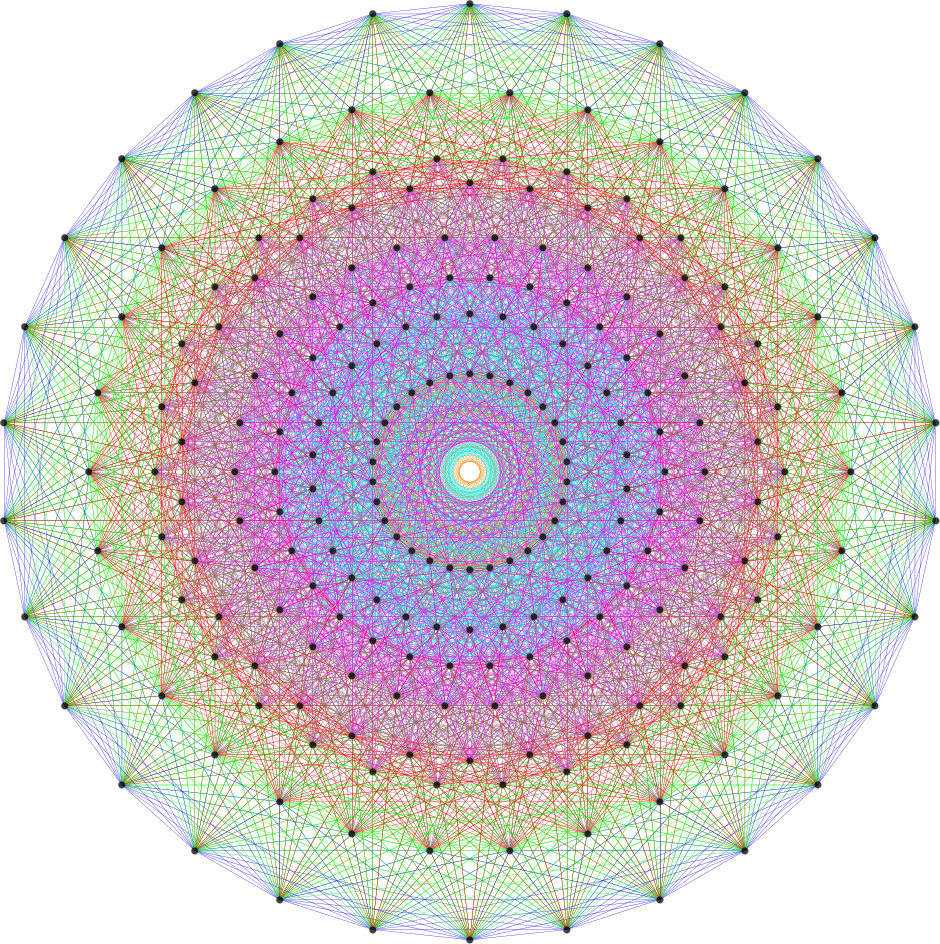Figure 4. E8 Coxeter plane projection of the 421 polytope.

This 4-dimensional projection of the 421 polytope is the compound of two 600-cells. The outer four red rings are four 30-gons with (4×30=120) vertices of a 600-cell. The inner four blue rings denote the 120 vertices of a smaller 600-cell inside the larger one. A 30-gon is the Petrie polygon for the 600-cell. Its interior angle is 168°. Each isosceles triangular sector of the triacontagon has a base angle of 84°.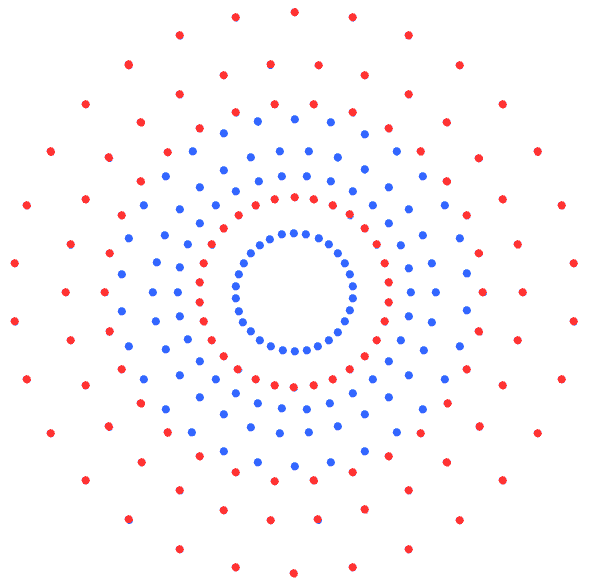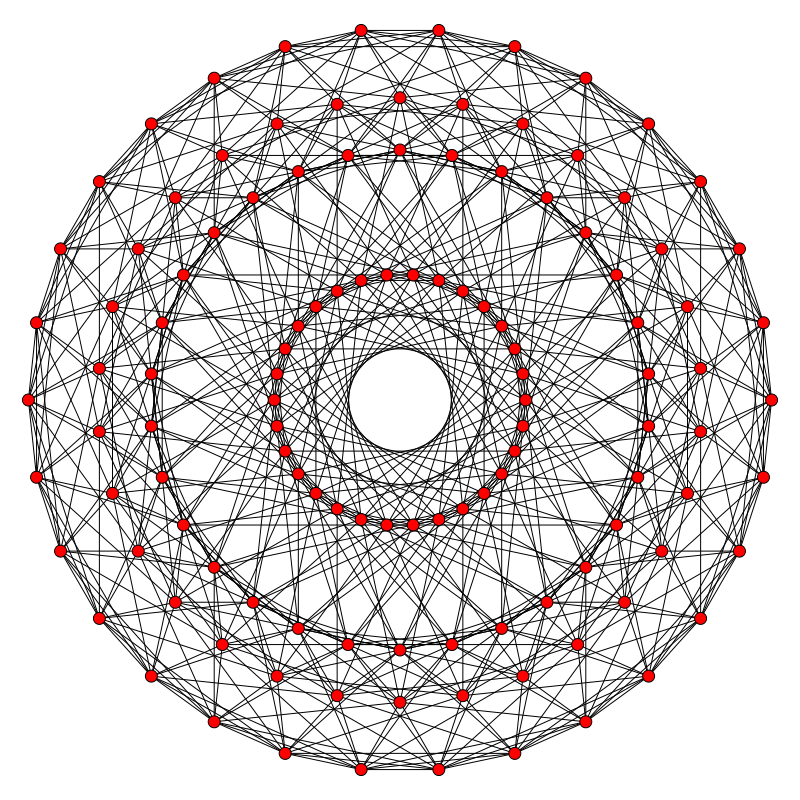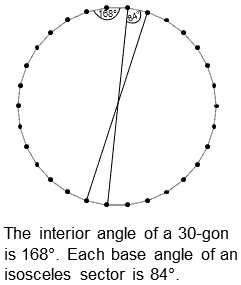Eight 30-gons in the E8 Coxeter plane projection of the 421 polytope. The 4-dimensional Coxeter plane projection of the 600-cell has four 30-gons. The interior angle of the 30-gon is the holistic parameter 168.

This 84:84 division is characteristic of the holistic parameter 168, being found in numerous contexts throughout this website. That this number appears naturally in the projected geometry of the 421 polytope, which mathematicians know represents the 240 roots of E8, is strong evidence that the UPA is a state of the E8×E8 heterotic superstring. Indeed, the same division manifests in the (120+720=840) vertices & edges in each of the two 600-cells whose compound is the E8 Coxeter plane projection of the 421 polytope! Just as the internal angle of the triacontagon is (84+84=168°), so the number of vertices & edges in the two 600-cells is (840+840=1680). See the section Triacontagon for how 30-gons embody the superstring structural parameters 840 and 1680 paranormally ascertained by C.W. Leadbeater.

As found earlier, the 1200 faces of the 600-cell contains 9240 points, lines & triangles when constructed from Type A triangles. They comprise the original 840 vertices & edges and 8400 new points, lines & triangles generated from the seven points, lines & triangles inside each face. The 2400 faces of two such polytopes comprise (840+840=1680) vertices & edges and (8400+8400=16800) new points, lines & triangles (4800 points or lines and 3600 triangles, or 4800 points or triangles and 3600 sides). What this means is that 8400 geometrical elements are needed to construct the faces of each 600-cell from simple triangles in a way that is consistent with the construction of other polytopes from triangles — a requirement which demands that all polygonal 2-faces be divided into their sectors. They comprise 1×1200 points, 3×1200 lines and 3×1200 triangles, that is, 7×1200 geometrical elements. Many previous articles have shown that this inner form embodies the numbers 840 and 1680, and now we encounter these very numbers in the numbers of vertices & edges making up the two concentric 600-cells whose 240 vertices are root vectors of E8. As these numbers are the numbers of circular turns in, respectively, an outer or inner half of a helical whorl and a whole whorl, the inevitable conclusion to be drawn is that the UPA is, indeed, an E8×E8 heterotic superstring the symmetry of whose forces is described by E8. In other words, the 421 polytope embodies not only the 240 roots of E8 but also the number of circular turns in the 10 helical whorls along which the 240 E8 gauge charges are spread. Each of the 16800 circular turns would correspond to one of the 16800 geometrical elements in the faces of the two 600-cells generated by their construction from basic triangles. One of the 600-cells with 120 vertices and 840 vertices & edges would correspond to the outer half of the UPA as its 10 whorls make 25 revolutions, the 2½ revolutions in each helical whorl comprising 840 turns, and the other 600-cell with 120 vertices would correspond to its inner half as its 10 whorls make another 25 revolutions around its axis. The appearance of the numbers 840 and 1680 in the two 600-cells making up the 421 polytope is strong evidence that the UPA is an E8×E8 heterotic superstring. The appearance of the numbers 8400 and 16800 in their construction from Type A triangles is, so to speak, the “icing on the cake,” discrediting the possibility of coincidence beyond all reasonable doubt. They refer to both the geometrical composition and the boundary yods of the two 600-cells, for — as we found earlier — 8400 yods lining tetractyses in addition to those on edges are needed to construct the faces of each 600-cell, so that (8400+8400=16800) new boundary yods are needed to create the faces of both 600-cells making up the 421 polytope.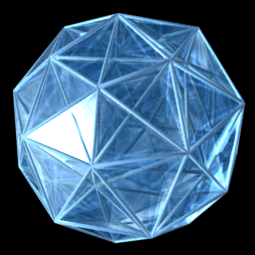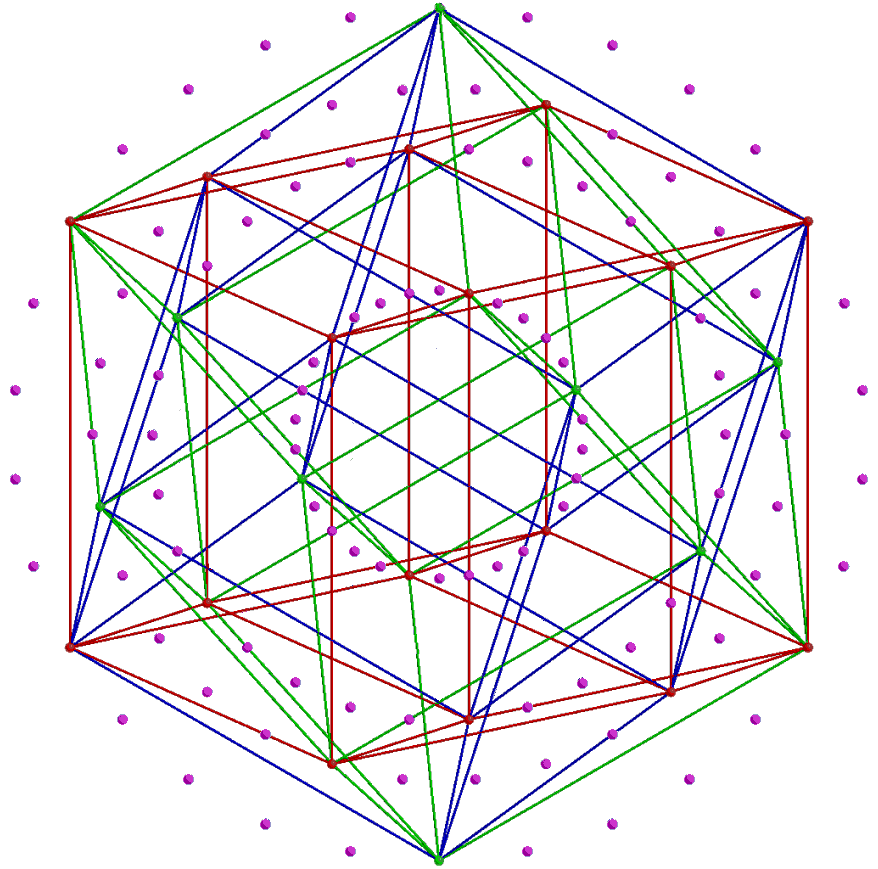Figure 5. Zome model of the 600-cell as a compound of five 24-cells. (Credit: http://homepages.wmich.edu/~drichter/five24cells.htm). 3-dimensional projection of the 600-cell. (Credit: "600-cell" by Jason Hise, uploaded to Wikipedia.org.). A 24-cell in the 600-cell. Shown are the ×32 red, blue & green edges of 3 hypercubes making up the 24-cell.

It is known to mathematicians that the 120 vertices of a 600-cell can be portioned into those of five disjoint 24-cells, each with 24 vertices (Fig. 5). This compares with the fact that the dodecahedron with 20 vertices is a set of five distinct tetrahedra. The two 600-cells comprise 10 disjoint 24-cells. This 10-fold character was shown in Article 53 to be a natural property of sacred geometries, indicating that it is the geometrical basis of either the 10 whorls of the UPA or its 10 half-revolutions. A similar property now emerges in the geometry of the 421 polytope as the convex hull of two 600-cells. As we saw earlier, it has its striking counterpart in the inner form of 10 overlapping Trees of Life as the 10 sets of 24 yods that are either centres of the (70+70) enfolded polygons or shared with these Trees. This 10-fold pattern in the E8 lattice of 240 roots suggests that the whorl “carries” 24 E8 gauge charges corresponding to 24 roots that are represented by the 24 vertices of a 24-cell. The UPA would have 10 whorls because the two 600-cells consist of 10 disjoint 24-cells. Alternatively, a 24-cell could represent a half-revolution of all 10 whorls, the two 600-cells determining the outer and inner halves of the UPA, each of which comprises 2½ revolutions of 10 whorls around the axis of the UPA, the five half-revolutions being represented by the five 24-cells.Figure 6. The connection between the UPA/superstring, the 421 polytope and 4-polytopes.

The compound of two 600-cells that is the 4-dimensional projection of the 421 polytope is the 4-dimensional manifestation of this archetypal pattern exhibited by sacred geometries. Constructed from Type A triangles, it contains 4080 points, where

4080 = (24 + 25 + 26 + 27) + (28 + 29 + 210 + 211),

240 = 24 + 25 + 26 + 27

is the number of vertices of the two 600-cells and

3840 = 28 + 29 + 210 + 211

is the number of other corners of their triangles. The two 600-cells have 13200 sides of 11520 triangles, i.e., 11760 sides other than their 1440 edges, where 11760 = 1680×7. This is the number of turns in the seven helical minor whorls of the UPA. Each cell has 5760 triangles with 6600 sides, where 5760 = (1+2+3+4)12×22×32×42 and 6600 = 55×120. The number 55 is the sum of the first 10 integers and 120 is the sum of the first 10 odd integers after 1, showing how the Decad determines the number of straight lines constructing the 600-cell. The Decad also determines its number of edges because 720 yods surround the centre of a decagon with 2nd-order tetractyses as its sectors (see Fig. 9).

The 10×24 pattern characteristic of sacred geometries manifests in the subatomic world in the E8×E8 heterotic superstring as the 120 E8 gauge charges that are spread along the inner and the outer halves of the 10 whorls of the UPA, 12 charges per half-whorl (Fig. 6). The composition of each 600-cell representing the E8 charges carried by the inner or outer half of the UPA in terms of five 24-cells determines the five half-revolutions of each half. As 16800 = 240×70, it means that each E8 gauge charge manifests as 70 turns of a helical whorl. This is both the number of yods in the Tree of Life created from tetractyses and the number of corners of the (7+7) enfolded polygons of its inner form. As might be expected, each charge is a whole in itself and so must be represented by a Tree of Life whose 16 tetractyses are composed of 70 yods. The boundary of the 24-cell is composed of 24 octahedral cells with six meeting at each vertex, and three at each edge. Together they have 96 triangular faces, 96 edges, and 24 vertices. The vertex-first parallel projection of the 24-cell into 3‑dimensional space has a rhombic dodecahedral envelope. Twelve of the 24 octahedral cells project in pairs onto six square dipyramids that meet at the centre of the rhombic dodecahedron. The remaining 12 octahedral cells project onto the 12 rhombic faces of the rhombic dodecahedron.

The superstring structural parameter 1680 and its connection to the 240 roots/gauge charges of E8 manifest in another context. The sphere in 3-dimensional space R3 has a 2-dimensional surface S2. In (n+1)-dimensional space Rn+1, the n-sphere Sn with radius r is the set of points {x1,x2,…xn+1} equidistant from a point {c1,c2,…cn+1} such that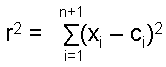A question that has occupied mathematicians for many years is: how many n-spheres can be packed in Rn+1 so as to touch a central n-sphere? This is called the “kissing number problem,” the number K(n) being called the “kissing number.” In 1-dimensional space, the 0-sphere is a point and the answer is 2 because a point at the middle of a finite straight line is equidistant from the two points ends of this line. In 2-dimensional space, the 1-sphere is a circle and six similar circles can be arranged at the corners of a hexagon so as to touch a circle of the same radius at its centre. In 3-dimensional space, K(3) = 12, although it took until 1953 to prove this rigorously. In 4-dimensional space, K(4) = 24 (first proved in 2003 by Oleg Musin). The densest lattice packing of spheres in this space is for 24 3-spheres centred on the vertices of a 24-cell. It explains why the 600-cell with 120 vertices can be regarded as five disjoint 24-cells. The problem is still unsolved for general n>4, although various upper and lower limits for K(n) have been derived for certain value of n. However, the kissing number problem is exactly solvable for spheres in eight and 24 dimensions. It was first proved in 1979 that K(8) = 240. Amazingly, the number of 7-spheres that can touch a central sphere in 8-dimensional space is the same as the number of vectors of the 8-dimensional root lattice space of E8! Not only that, their centres coincide with the 240 lattice points! A point in 8-dimensional space has the spherical coordinates (r, φ1, φ2, φ3, φ4, φ5, φ6, φ7). The positions of points on 240 7-spheres require (240×7=1680) angular coordinates to specify them. This is the number of 1st-order spirillae in a whorl of the UPA that Leadbeater painstakingly counted 135 times . It is yet another way in which this superstring structural parameter appears in the group mathematics of E8×E8 heterotic superstrings.

Of course, the author does not claim that this property (or, indeed, the properties of the 421 polytope as the convex hull of two 600-cells discussed earlier) is the explanation of the string winding number 1680. This because the number refers to a property of spheres packed in a special, 8‑dimensional lattice, whereas in the context of the UPA, it refers to the number of times a closed curve (whorl) winds around a 2-torus in 3-dimensional space. The crucial point is that it is highly unlikely that it could be just by chance that this number with a paranormal provenance appears naturally twice in a narrow context connected to the group mathematics of superstrings, namely, the 421 polytope representing the root lattice of E8 as the convex hull of two 600-cells with 1680 vertices & edges and the 240 root vectors of E8 specified by 1680 angular coordinates. So any criticism that all these mathematical facts on their own do not explain the 1680 turns in a helical whorl of the UPA misses the point, for it is not being claimed here that these facts on their own do explain them, merely that they are a visible component of the complete explanation to be provided by the as yet undiscovered M-theory. The argument against coincidence has even more force vis-à-vis the UPA’s structural parameters 840, 8400 & 16800, which we have seen manifest uniquely in this polytope. The conclusion is inescapable that the UPA is a state of the E8×E8 heterotic superstring and that both its space-time structure and the symmetry of its forces are laid out in the 4-dimensional sacred geometries discussed in this section. The difference between all previous discussions of the embodiment of these numbers and this one is that mathematicians have known for at least a century how the polychorons and the 421 polytope are related to the five exceptional Lie groups, the largest of which was established thirty years ago by physicists Michael B. Green and John H. Schwarz as being relevant to one of the five types of superstrings. What has now emerged is the existence of an undeniable connection between these numbers of paranormal provenance and well-established facts in a branch of mathematics that is too detailed to be dismissed as fortuitous.

The Tetrad determines the superstring structural parameter 16800
According to Table 2, 14400 geometrical elements surround the centre of a 600-cell constructed from Type A triangles. Two 600-cells contain 28800 geometrical elements surrounding their centres, where 28800 = 1!×2!×3!×4!(13+23+33+43). According to Table 3, their centres are surrounded by 42000 yods, where 42000 = 1680×25. Noting that 168 = 132 − 1 = 3 + 5 + 7 +... + 25, i.e., it is the sum of the first 12 odd integers after 1, the superstring structural parameter 1680 is the sum of the 12 integers 30, 50, 70, ... 250 that can be assigned to the 12 yods on the boundary of a Type A square,
which has 25 yods, whilst 4200 is the sum of the same integer 1680 assigned to all its yods:If the sceptic thinks that it is just coincidence that the number of vertices & edges in two 600-cells should be the structural parameter of the basic unit of matter that C.W. Leadbeater claimed to remote-view, despite their being connected to the 421 polytope representation of the roots of the symmetry group E8 in E8×E8 heterotic superstring theory, then — to remain consistent — he has to accept that it is also just a matter of chance that the square should simultaneously generate this number and the yod population of the two 600-cells. How many such miraculous coincidences is he willing to accept before he grudgingly admits that a profound, mathematical design embodied in the UPA is being revealed here? Any argument based upon chance that he uses to justify his scepticism is untenable, given the degree of correlation established in this article between the features of the UPA, the geometry of the two 600-cells as the 4-dimensional projection of the 421 polytope and the five-fold distribution of their vertices in 24-cells.

The Pythagorean integers 1, 2, 3 & 4 determine the 2400 faces of the two 600-cells because

 11 12 13 14 43 44 21  21 22  22 23  23 24  24 33  33 34  34 2400 = 31  31  31 + 32  32  32 + 33  33  33 + 34  34  34 + 23  23  23 + 24  24  24 41  41  41  41 42  42  42  42 43  43  43  43 44  44  44  44 13  13  13  13 14  14  14  14

They determine the number 1680 because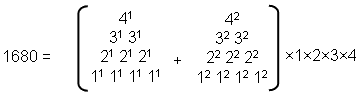They determine the number 16800 because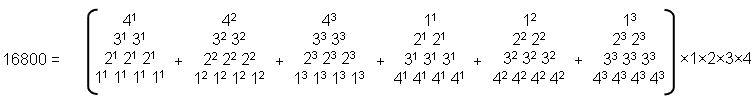The holistic 72:168 division in the two 600-cells making up the 240 vertices of the 421 polytope
As additional evidence of the holistic nature of the compound of two 600-cells, it will be next proved that the yod composition of their faces displays the 72:168 division characteristic of holistic systems (see The holistic pattern).

Table 3 indicates that the 1200 faces of the 600-cell contains 13560 yods. Its 720 edges comprise (120 + 2×720 = 1560) yods. (13560−1560=12000) new yods are needed to turn the faces into Type A triangles. Of these, 3600 hexagonal yods are at the centres of the (3×1200=3600) tetractyses, leaving 8400 new yods on their sides. Therefore, 12000 = 3600 + 8400. (2×12000=24000) new yods are needed to construct the 2400 faces of the two 600-cells. They contain (2×3600=7200) hexagonal yods at the centres of the 7200 tetractyses and (2×8400=16800) new yods on their sides. Hence:

24000 = 7200 + 16800.

Ignoring the Pythagorean double factor of 100 originating from the fact that the two 600-cells have 2400 (=24×100) faces, we see that the pair of two 600-cells exhibits the 72:168 division that is found in all other sacred geometries. Moreover, the 36:84 division differentiating (apart from a factor of 100) between central hexagonal yods and boundary yods is exactly the same as the division of the 120 yods on the boundaries of each separate set of seven enfolded polygons into 36 corners and 84 hexagonal yods (Fig. 7).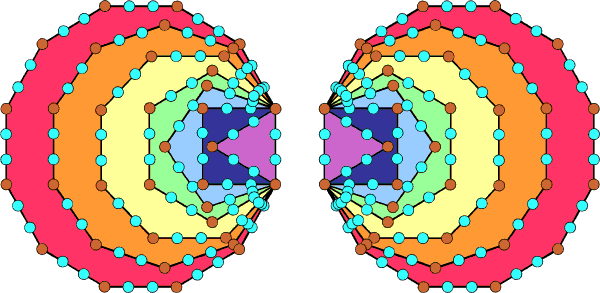Figure 7. The 120 yods lining the sides of each set of 7 enfolded polygons consist of 36 brown yods at their corners and 84 turquoise hexagonal yods.

Article 62 discusses how other sacred geometries manifest this pattern in the holistic parameter 240. The fact that it is revealed in the very pair of two 600-cells that generates the 421 polytope when they are constructed from tetractyses is truly remarkable evidence of the archetypal nature of this polytope as sacred geometry. In terms of the 240 roots of E8, this division is between the 72 roots of its exceptional subgroup E6 and its 168 remaining roots. Here is the sacred geometrical counterpart of this property.

The holistic 192:192 division in the two 600-cells
We found in (2) above that the centre of a 600-cell is surrounded by 1920 corners of sectors in its 1200 faces and 720 interior triangles that are not vertices. Therefore, (192+192=384)×10 points other than vertices surround the common centre of two 600-cells. As

 12 22 32 385 = 42 52 62 72 82 92 102 ,

384 is the sum of the squares of 2-10, so that 1920 = 5×(22 + 32 + ... + 102). This means that the sum of the 90 squares of 2-10 arranged in a 10-fold array surrounding the central square of 1 (Fig. 8) is 3840 — the number of corners other than vertices of the 7200 sectors of the 2400 faces of two 600-cells. This demonstrates the remarkable way in which the Decad determines the number of points other than vertices that surround the common centre of the two 600-cells.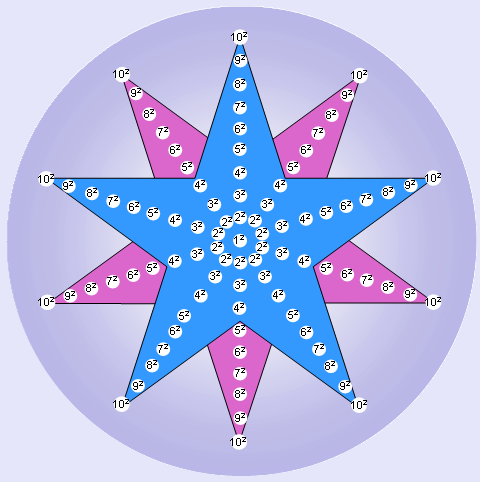Figure 8. Each pentagram array of the squares 22-102 sums to 1920. This is the number of corners of triangles in each 600-cell that are not its vertices or, alternatively, the number of its external and internal triangles when both types are simple triangles.

Alternatively, if the 120 vertices of the 600-cell are joined to its centre, creating the sides of triangles, and the internal triangles and the faces treated as simple triangles, then there are (1200+720=1920) triangles and 1920 lines & triangles in its faces. It has (120+720=840) internal sides & triangles, as well as (720+120=840) external & internal lines and (120+720=840) points & lines in its faces. So there are three possible sets of 840 geometrical elements: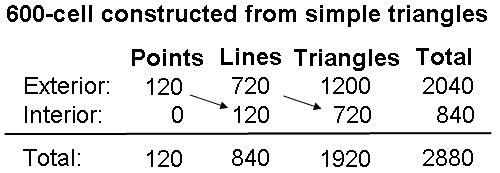Assuming that their sets of 840 elements are compounded in the same way, the two 600-cells sharing the same centre are composed of four possible sets of 1680 geometrical elements:

1. (840+840) points & lines in their 2400 faces;
2. (840+840) external & internal lines;
3. (840+840) internal lines & triangles;
4. (840+840) points & triangles.

Given that the compound of two 600-cells is composed of 1680 geometrical elements in four different ways, there can be no doubt whatsoever that it embodies the paranormally obtained superstring structural parameter 1680! It does not need its construction from Type A triangles to demonstrate that — its own, unreconstructed geometry suffices. But the latter is required to show that the compound embodies the numbers 8400 and 16800 as well. Notice that even a single 600-cell can embody the number 1680, for its faces are composed of 840 points & lines, whilst its interior has 840 lines & triangles. Alternatively, it has 840 lines and 840 points & internal triangles. The question now arises: which of all these possibilities is the correct geometrical analogue of the 1680 turns in a helical whorl of the UPA? To answer this question, it must be pointed out that, whilst necessary, it is not sufficient that a parameter of holistic systems be embodied in a geometry for the latter to count as sacred. True parameters of holistic systems are not isolated; they are also interconnected, forming mathematical patterns that are always the same from one holistic system to another. Study of these systems has shown that one such pattern is the sum:

192 = 24 + 168

(or a pattern with 10 times these numbers). A holistic parameter may be present in a sacred geometry in more than one way. Its "correct" way will be that which is consistent with the holistic patterns that the geometry displays. In the case of the 600-cell constructed from simple triangles, there are (720+1200=1920) lines & triangles in its faces and (720+1200=1920) triangles in its faces and interior. In neither case, however, does this number include the number 240. This appears only as the sum of the 120 vertices and the 120 internal lines, but in each case the number 120 is also part of the number 840 instead of being separate from it, which it needs to be in order to create the sum: 1920 = 240 + 1680. This holistic pattern only manifests in the pair of 600-cells, which contains 240 points (vertices) and 1680 lines (edges & internal lines), i.e., 1920 points & lines. As we have found that sacred geometries embody the numbers 384 and 192 (or 10 times these numbers) as:

384 = 192 + 192,

(see The holistic pattern and #19, #49, #58 & #59 in Wonders of correspondences), it indicates that the truly complete, holistic system exhibiting the division: 3840 = 1920 + 1920 is one in which the compound is replicated, i.e., there should be two 421 polytopes, each with 240 vertices determining the 240 roots of E8. But this is, precisely, the E8×E8 heterotic superstring theory, which this website demonstrates is encoded in the inner Tree of Life, the five Platonic solids and the disdyakis triacontahedron! Hence, this particular superstring theory is the simple consequence of holistic systems having two suitably defined "halves", each embodying the number 192 (or 1920). The correct way of seeing how the superstring structural parameter 1680 is embodied in either the 600-cell or two 600-cells composed of simple triangles is the one that is consistent with both the sums: 1920 = 240 + 1680 and 1680 = 840 + 840. The only one that is consistent with them is a compound of two 600-cells with 240 points, 1680 lines and 1920 points & lines, i.e., each 600-cell with 840 sides of external and internal simple triangles represents half a helical whorl with 840 turns. This is consistent with the conclusion reached in Article 62 that, in terms of its vertices, the two 600-cells composed of Type A triangles represent the outer and inner halves of a UPA/E8×E8 heterotic superstring, the 120 vertices in each denoting 120 E8 roots/gauge charges that are spread along either half, whilst each of the five half-revolutions of the 10 whorls in each half is represented by one of the five disjoint 24-cells that make up a 600-cell. The division: 840 = 120 +720 observed for the sides of exterior and interior triangles in each 600-cell is the exact counterpart of what is found in the inner & outer Trees of Life, where 120 yods making up half of the 1-tree constructed from Type A triangles can be associated with the 720 yods that surround the centres of each set of seven separate Type B polygons (see Fig. 7 in #1 of this section). That the superstring divides into an inner and an outer half due to this 120:120 division of vertices/E8 gauge charges is confirmed by the fact that, when the faces and interior triangles of each 600-cell are Type A triangles instead of simple triangles, (7×1200=8400) geometrical elements are added in the 1200 faces of each 600-cell. In other words, these elements are the counterpart of the 8400 helical turns in the outer or inner halves of the UPA.

The Decad determines 120, 1200, 720 & 840
The ancient Pythagoreans regarded 2 as the first true integer because they taught that the number 1 (the Monad) was not a number but the source of all numbers, including integers. They would have treated the number 11, the tenth integer after 1, as the tenth true integer and 3 as the first true odd integer. As 112 − 1 = 121 − 1 = 120 = 3 + 5 + 7 +... + 21, the Decad determines the 120 vertices of the 600-cell arithmetically as the sum of the first 10 odd integers. It also determines this number in a geometrical way because the centre of a Type B dodecagon is surrounded by 120 points, lines & triangles and the dodecagon is the 10th type of regular polygon. The Decad determines its 1200 faces because 1200 = 10×120 = 10(3+5+7+... +21) = 30 + 50 + 70 +...+210. The tenth prime number is 29. As 292 − 1 = 840 = 3 + 5 + 7 +... + 57, the number 840 is the sum of the first 28 odd integers after 1. Therefore,

840 − 120 = 720 = 23 + 25 + 27 +... + 57,

i.e., 720 is the sum of 18 odd integers. The Decad determines arithmetically both the number of vertices in the 600-cell and its 840 vertices & edges. It determines the 720 edges as well because there are 720 yods surrounding the centre of a decagon whose sectors are 2nd-order tetractyses (Fig. 9).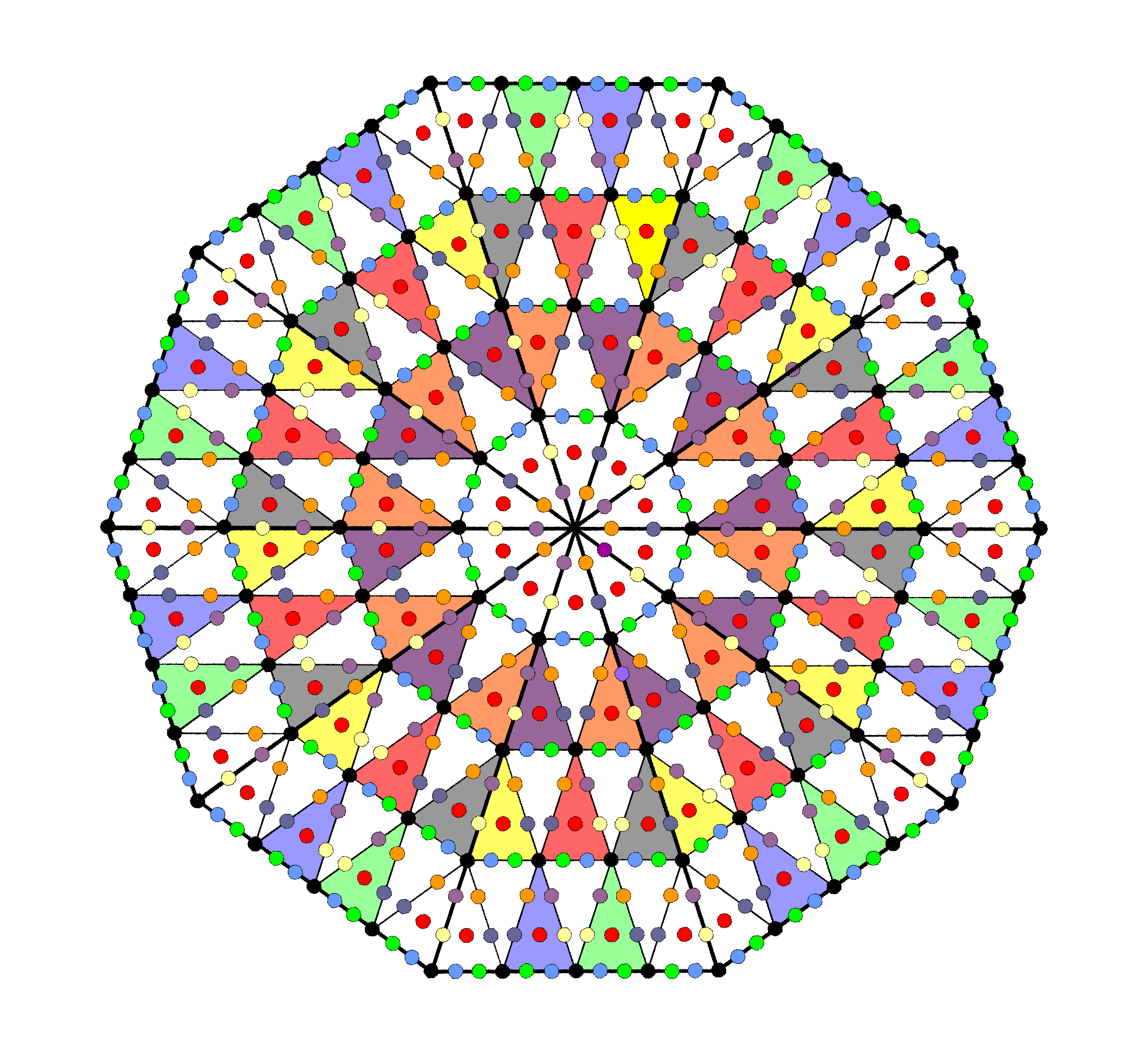Figure 9. 720 yods surround the centre of a decagon whose sectors are 2nd-order tetractyses.UPA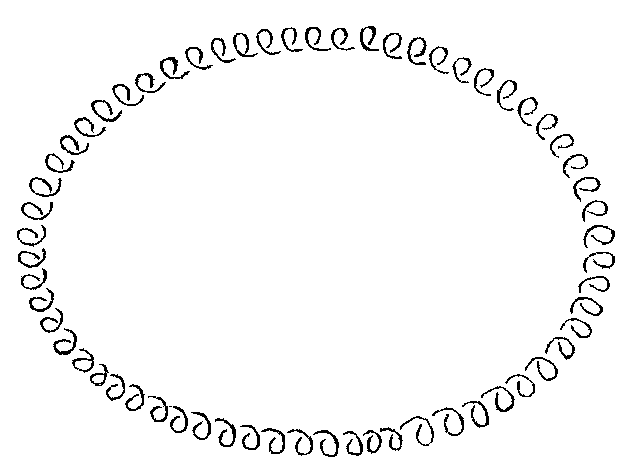whorl The UPA is a set of 10 closed curves, or "whorls." Each whorl is a closed helix with 1680 circular turns. Each whorl winds 5 times around the axis of spin. The 10 whorls make 50 revolutions around this axis. Each whorl has 168 turns in a half-revolution. Each whorl has 336 turns in one revolution. The 10 whorls have 3360 turns in one revolution. The 10 whorls have 1680 turns in a half-revolution. The inner half of each whorl winds 2½ times around axis. The 10 whorls have 8400 turns in inner/outer half of UPA. Each whorl has 840 turns in inner/outer half of UPA3-d projection of the rotating 600-cell.3-d projection of the rotating 600-cell. 120 vertices→120 roots of E8. (120+720=840) sides of (1200+720=1920) triangles. 1680 hexagonal yods line the sides of the 1920 tetractyses. 120 vertices→120 roots of E8. (120+720=840) sides of (1200+720=1920) triangles. 1680 hexagonal yods line the sides of the 1920 tetractyses.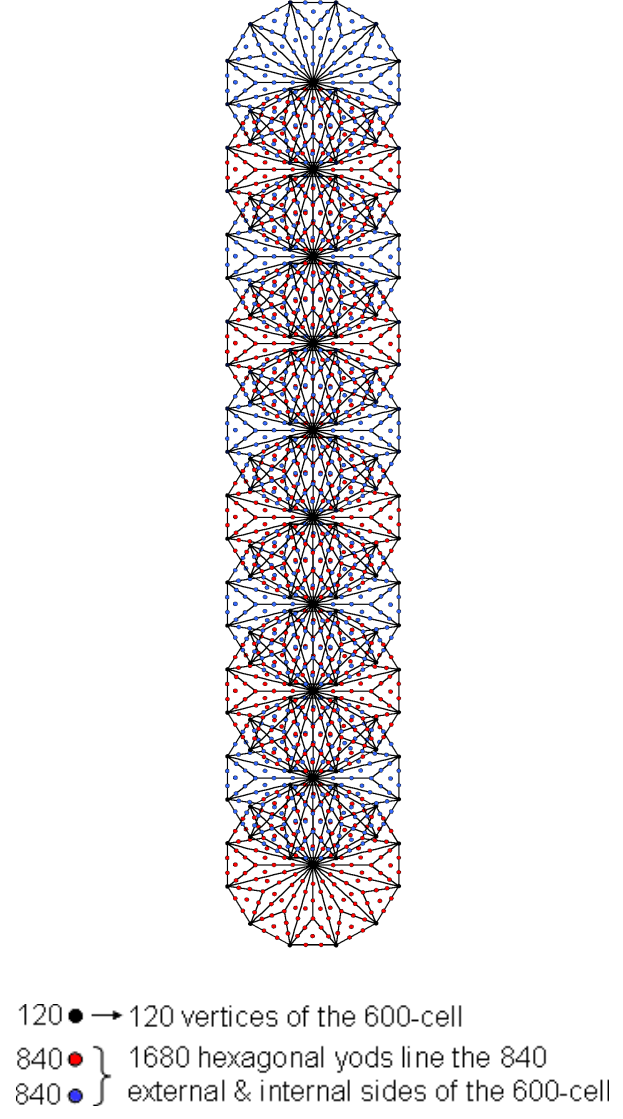The holistic 168:168 division in the two 600-cells
When the 600-cell is composed of simple tetractyses, (720×2=1440) hexagonal yods line sides of the 1200 tetractyses in its faces and (120×2=240) hexagonal yods line the 120 internal sides of the 720 tetractyses in its interior. Therefore, (1440+240=1680) hexagonal yods line the (720+120=840) sides of its (1200+720=1920) tetractyses. The superstring structural parameter 1680 recorded by C.W. Leadbeater when he remote-viewed the UPA is the number of hexagonal yods in a single 600-cell that correspond (in terms of the formal correspondence between yods and Sephiroth) to the six Sephiroth of Construction above Malkuth. Here is yet more confirmation of the superstring nature of the UPA. The pair of 600-cells has (1680+1680=3360) hexagonal yods on the 1680 sides of their (1920+1920=3840) tetractyses. This is the number of turns in one revolution of the 10 whorls. Once again, we see that each 600-cell is representing all 10 whorls, but this time a half-revolution of them instead of five half-revolutions. See The holistic pattern for examples of sacred geometries displaying the 168:168 or 1680:1680 divisions. The remarkable ways in which polygonal numbers and sacred geometries represent the superstring structural parameter 3360 (number of turns in one revolution of the 10 whorls of the UPA) are shown here. Notice, finally, how the pair of 600-cells manifests the 192:192 division of the holistic parameter 384 as the 1920 tetractyses in each 600-cell.

The holistic 120:720 division in the two 600-cells and in sacred geometries
Figure 7 in #1 displays how the number 1680 is embodied in 1. the disdyakis triacontahedron, 2. the 1-tree & the two sets of 7 separate Type B polygons, and 3. the first four Platonic solids. Their geometrical or yod compositions conform to the pattern:

1680 = 720 + 240 + 720.

This pattern manifests in a single 600-cell composed of simple triangles as the 720 edges, (120+120=240) vertices & internal sides and 720 internal triangles. It appears in two 600-cells as their 240 vertices and their pair of 720 edges. Let us examine these sacred geometries in more detail in order to demonstrate how the holistic 120:720 division exhibited by the 600-cell in both its faces and its interior and by two 600-cells in their vertices & edges manifests in each half of these sacred geometries.

Disdyakis triacontahedron
The polyhedron has 62 vertices, 180 edges & 120 triangular faces. Surrounding an axis passing through two diametrically opposite vertices are 360 (=36×10) geometrical elements comprising 60 vertices, 180 edges, i.e., 240 vertices & edges, and 120 triangles. Joining its vertices to its centre creates internal triangles which, when Type A, are composed of 180 points, (60 + 3×180 = 600) lines & (3×180=540) triangles surrounding the axis. Hence, (60+180=240) points, (180+600=780) lines & (120+540=660) triangles surround the axis. Each half of the disdyakis triacontahedron is composed of 120 points, 390 lines & 330 triangles. i.e., 120 points and 720 lines & triangles. Therefore, the 1680 geometrical elements surrounding the axis consist of two sets of elements, each comprising 840 elements made up of 120 points and 720 lines & triangles. Each half of the disdyakis triacontahedron is the 3-dimensional analogue of a 600-cell with 120 vertices & 720 edges.

Outer & inner form of 1-tree
Constructed from 19 Type A triangles, the 1-tree contains 251 yods. Of these, 11 are corners of triangles that coincide with Sephiroth, leaving 240 yods generated by this transformation. The seven regular polygons making up each half of its inner form contain 727 yods when they are Type B. 720 yods surround their centres. It was shown here that the 240 yods in the 1-tree consist of 10 sets of 24 yods. Yods in triangles that are on the left or the right of the central Pillar of Equilibrium can be associated with the yods in the seven regular polygons on that side. The yods that line this pillar as the axis of symmetry of the 1-tree can be divided into two sets containing the same number. Of course, unlike the yods in each set of polygons, these central yods are not mirror images of each other. However, one set can be associated with the left-hand set of polygons and the other set associated with the right-hand set. This means that 120 yods in the 1-tree can be associated with each set of polygons. The 1680 yods can be divided into two sets of (120+720) yods.

When the interior triangles of a 600-cell formed by joining its centre to its vertices are simple triangles, it has (1200+720=1920) triangles with (120+720=840) sides. The compound of two 600-cells has (1920+1920=3840) triangles with (840+840=1680) sides. This shows how the pair of 600-cells embodies the superstring structural parameter 1680, the 840 sides of the 1920 triangles in each 600-cell corresponding to the 840 turns in an outer or inner half of the UPA/superstring. The same 120:720 division appears in the inner form of 10 overlapping Trees of Life whose (70+70) enfolded Type B polygons are divided into their sectors: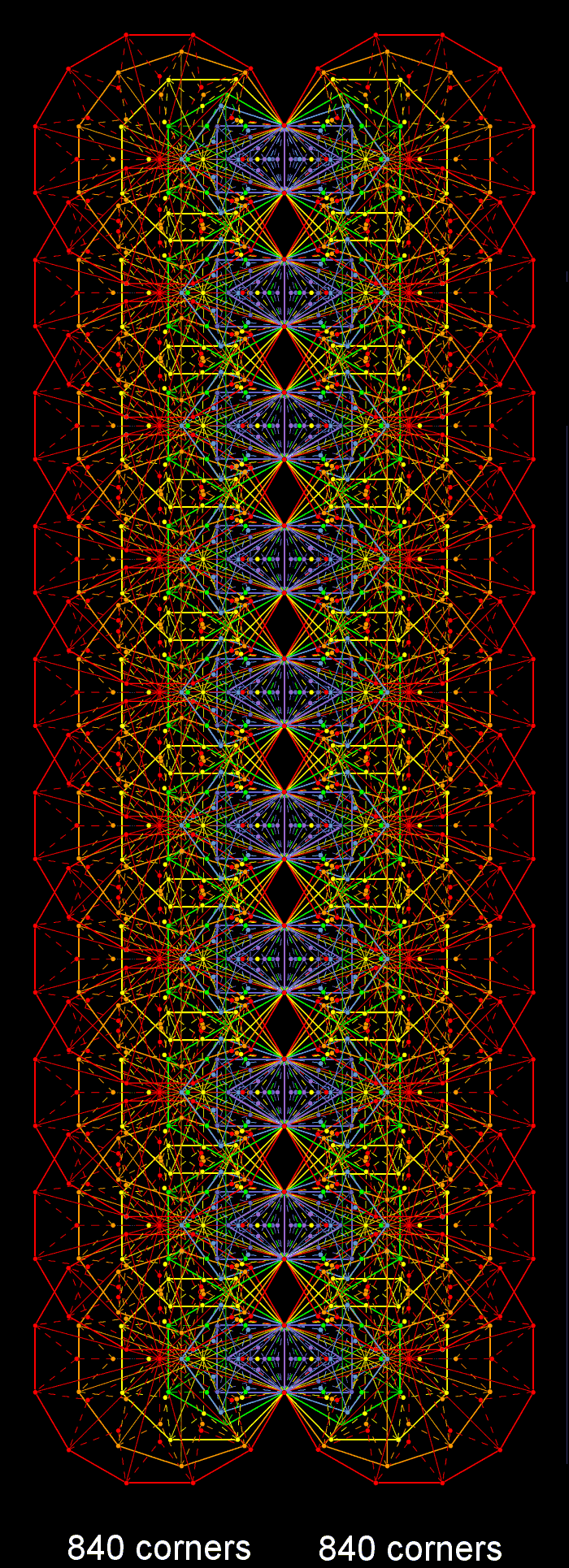The 3n triangles in a Type B n-gon have (2n+1) corners. The 36 triangles in a Type B dodecagon (n=12) have 25 corners, of which 13 are corners of its 12 sectors/Type A triangles. 12 corners are associated with each dodecagon. Noting that the triangle occupies one of the sectors of the hexagon, a corner of the triangle coincides with the centre of the hexagon and that a corner of a pentagon coincides with the centre of the decagon, the number of corners in the (47×3=141) triangles making up the 47 sectors of the 7 enfolded Type B polygons = ∑(2n+1) − 6×2 − 1 − 1 − 1 = 2×48+ 7 − 12 − 3 = 88. Three of these coincide with Sephirothic corners of triangles in the outer Tree of Life, leaving 85 intrinsic corners, where

85 = 40+ 41+ 42+ 43.

Of these, 84 are associated with each set of 7 enfolded polygons. The (7+7) enfolded Type B polygons have (84+84=168) corners of 282 triangles that are unshared with the outer Tree, where 282 is the number value of Aralim, the Order of Angels assigned to Binah. The 84 corners consist of the 12 corners of the 12 sectors associated with the dodecagon, leaving 72 corners. Associated with the 1410 triangles in each set of 70 Type B polygons enfolded in 10 overlapping Trees of Life are 840 intrinsic corners. They consist of the 120 corners of the sectors of the 10 dodecagons and 720 other corners. This is how the 120:720 division characteristic of sacred geometries manifests in the inner form of 10 Trees of Life. Both sets of polygons enfolded in 10 Trees have 2820 triangles with 1680 corners unshared with these Trees:

1680 = 120 + 720 + 120 + 720 = 240 + 1440.

Each half of the inner form of 10 Trees has 840 corners. They correspond to the 840 turns in an outer or inner half of a whorl of the UPA/superstring, whose 10 space-time dimensions are represented by 10 Trees. Alternatively, each Tree represents a whorl (or, rather, one of its 10 half-revolutions) and the 10 Trees map the half-revolution of all 10 whorls of the UPA.

Correspondence with the compound of two 600-cells

 70 Type B polygons enfolded in 10 Trees of Life 600-cell 120 corners associated with 120 sectors of the 10 dodecagons; → 120 vertices/sides of internal triangles; 720 intrinsic corners of triangles in 70 polygons; → 720 edges; 840 intrinsic corners associated with 1410 triangles in 70 polygons; → 840 vertices & edges (or 840 sides of 1920 triangles); 24 corners associated with dodecagons enfolded in 2 Trees; → 24 vertices of 24-cell; 120 corners associated with 5 pairs of dodecagons enfolded in 10 Trees; → 120 vertices of compound of 5 24-cells.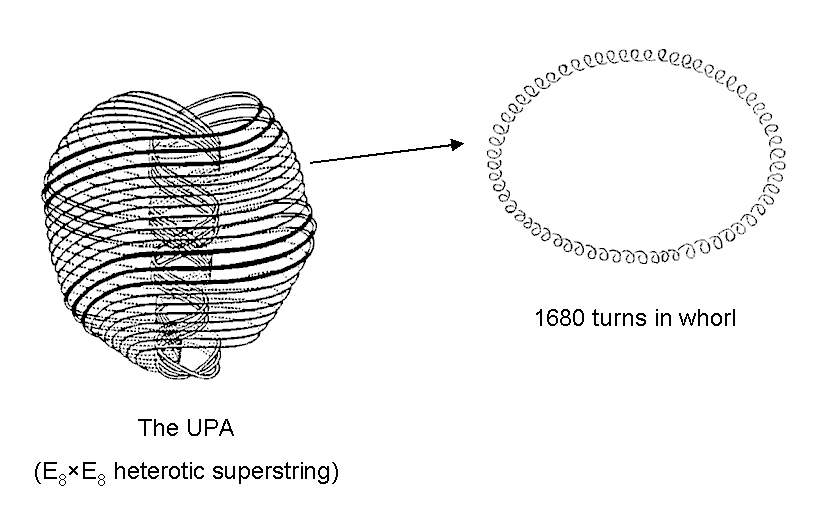600-cell600-cell Each of the 10 whorls of the UPA twists 5 times around its axis of spin, 2½ times in its outer half and 2½ times in its inner half. Each half of a helical whorl has 840 circular turns. Each member of the compound of two 600-cells that is the Petrie projection of the 421 polytope has 840 vertices & edges (or sides of external and internal triangles). Their superstring manifestation is the 840 turns in the outer or inner halves of each whorl of the UPA/E8×E8 heterotic superstring.

First four Platonic solids
How the tetrahedron, octahedron, cube & icosahedron embody the number 1680 is calculated here. When their faces and interiors are constructed from Type A triangles, the numbers of corners, sides & triangles that surround their axes passing through two opposite vertices are tabulated below in Table 2:

Table 2. Geometrical elements surrounding the axes of the Platonic solids.

 Platonic solid Corners Sides Triangles Total Tetrahedron 24 78 66 168 Octahedron 48 156 132 336 Cube 48 156 132 336 Icosahedron 120 390 330 840 Subtotal = 240 780 660 1680 Dodecahedron 120 390 330 840 Total = 360 1170 990 2520

The 1680 geometrical elements surrounding the axes of the first four Platonic solids comprise 240 corners and 1440 sides & triangles. Surrounding the axes of the first three Platonic solids are 120 corners and 720 sides & triangles, i.e., 840 geometrical elements. Similarly, 120 corners and 720 sides & triangles, i.e., 840 geometrical elements, surround the axis of the icosahedron. Amazingly, the first three regular 3-polytopes and the icosahedron display the same 120:720 division as the 600-cell, which is the 4-dimensional counterpart of the icosahedron, the fourth Platonic solid! The compound of two 600-cells is the four-dimensional counterpart of the first four Platonic solids, their (120+120=240) vertices corresponding to the (120+120) corners of triangles that surround the axes of the (first three+fourth) Platonic solids. Together with the disdyakis triacontahedron, they are the 3-dimensional and 4-dimensional blueprints for the E8×E8 heterotic superstring, which was paranormally described by Besant & Leadbeater over a century ago. The same pattern is displayed by the icosahedron and the dodecahedron because the latter is the dual of the former and has the same geometrical composition. This is not repeated in their 4-dimensional counterparts because the 120-cell (the 4-dimensional counterpart of the dodecahedron) has 600 vertices, not 120 vertices. It is, however, repeated for its 120 dodecahedra and 720 pentagonal faces. Just as the disdyakis triacontahedron is generated from the rhombic triacontahedron as a compound of the icosahedron and dodecahedron, so the 4-dimensional projection of the 240 vertices of the 421 polytope is the compound of two 600-cells. In this sense, it can be regarded as analogous to the disdyakis triacontahedron — a 3-polytope. What is crucial to recognize is that the highly mathematical, hyper-dimensional objects that are being discovered to underpin E8×E8 superstring physics have their exact parallels in the sacred geometries of mystical traditions and ancient philosophies. Why? Because they represent the same thing: the divine paradigm.

How the Godnames mathematically prescribe the 600-cell and the pair of two 600-cells
Kether: EHIEH = 21
The first six rows in Pascal's triangle that contain binary coefficients other than 1 comprise 21 such numbers that add up to 240. This is the number of vertices in the two 600-cells: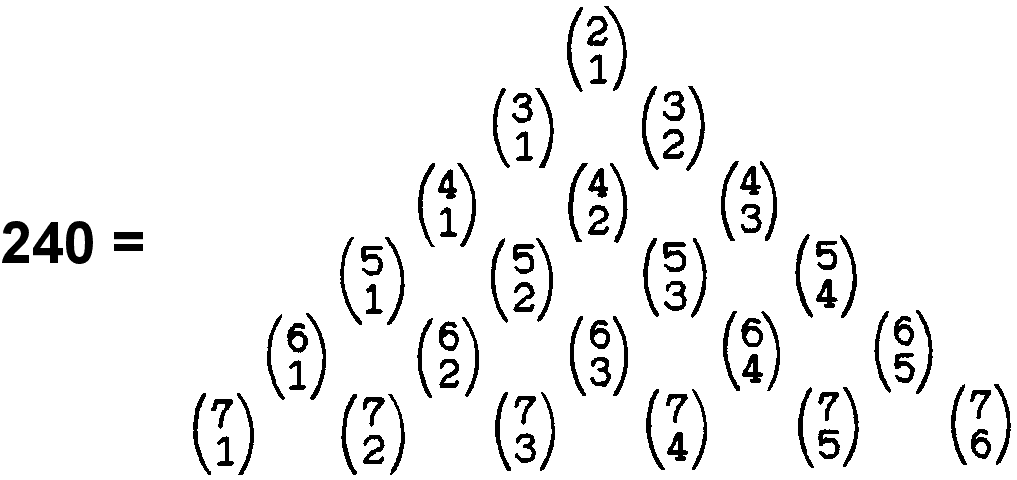The centre of the 600-cell constructed from Type A triangles is surrounded by 21000 (=21×10×10×10) yods (see Table 3 on #1).

Chokmah: YAH = 15
The sum of the first 15 positive integers is 120, i.e., it is the 15th triangular number. This is the number of vertices in the 600-cell. The sum of the first 15 even integers is 240, which is the number of vertices in the two 600-cells. The two 600-cells have 1680 vertices & edges. The sum of the 15 combinations of the four integers 21, 42, 63 & 84 (21 units apart) = 1680. The 15th prime number is 41, where 412 − 1 = 1680.

Binah: ELOHIM = 50
2040 corners of 5760 triangles surround the centre of the 600-cell. Each half has 2880 triangles with 1020 (=102×10) corners. 102 is the 50th even integer after 2. ELOHIM prescribes the form of the 600-cell by determining the number of the corners of its component triangles.

Chesed: EL = 31
The two 600-cells have 1680 vertices & edges. 1680 is the sum of the 31 combinations of the five integers 7, 14, 21, 28 & 35, which are 7 units apart. Construction of the faces of the 600-cell from Type A triangles adds (7×1200=8400) geometrical elements. The number 8400 is the arithmetic mean of the 31 square numbers 52, 102, 152155.2

Geburah: ELOHA = 36
The 1200 faces of the 600-cell have 3600 (=36×10×10) sectors. It has 720 edges, where 720 = 72×10 and 72 is the 36th even integer.

Tiphareth: YAHWEH ELOHIM = 76
The pair of 600-cells composed of simple triangles has (1200+1200=2400) triangles in its faces and (720+720=1440) internal triangles, making a total of 3840 (=384×10) triangles. 384 is the 383rd integer after 1, where 383 is the 76th prime number.

Netzach: YAHWEH SABAOTH = 129
Construction of the 2400 faces of two 600-cells from Type B triangles adds 16800 geometrical elements. As 168 = 132 − 1,

16800 = 168×102 = (132 − 1)102 = 1302 −102 = (2×65)2 − (2×5)2

= 4(652 − 52).

652 = 1 + 3 + 5 + 7 + 9... + 129

and

52 = 1 + 3 + 5 + 7 + 9.

Therefore,

16800 = 4(11 + 13 + 15 +... + 129).

The number 16800 is the sum of (4×60=240) odd integers, the smallest of which is 11, the 10th integer after 1, and the largest of which is 129, the 65th odd integer, where 65 is the sum of the first 10 integers after 1.

Hod: ELOHIM SABAOTH = 153
1560 (=156×10) yods line the 720 edges of the 600-cell when constructed from tetractyses or Type A triangles. 156 is the 153rd integer after 3, the first odd integer.

Yesod: EL ChAI = 49
Inside the 600-cell are 2160 triangles with 2280 (=228×10) sides, where 228 is the 227th integer after 1 and 227 is the 49th prime number. Two 600-cells have 2400 triangular faces. As 492 = 2401, 2400 = 492 − 1. This is the dimension of SU(49).

Malkuth: ADONAI = 65
The 600-cell has (120+720=840) vertices & edges. As 120 = 5! and 720 = 6!, 840 = 6! + 5!. These two integers make up the gematria number value of ADONAI. The 3600 triangular sectors of the 1200 faces of the 600-cell have 1320 (=132×10) corners, where 132 is the 65th even integer after 2. The 5760 triangles in its faces and interior have 6600 (=66×10×10) sides, where 66 is the 65th integer after 1.
ADONAI MELEKH = 155
The number of yods lining the 720 edges connecting the 120 vertices of the 600-cell = 120 + 720×2 = 1560 (=156×10). 156 is the 155th integer after 1.

The sum of the Godname numbers of the first six Sephiroth of Construction is 240 — the number of vertices of the 421 polytope denoting roots of E8, as well as the number of vertices of the two 600-cells:

240 = 21 + 26 + 50 + 31 + 36 + 76.

The 240 vertices of the 421 polytope can be constructed in two sets: 112 (22×8C2) with coordinates obtained from (±2, ±2, 0, 0, 0, 0, 0, 0) by taking an arbitrary combination of signs and an arbitrary permutation of coordinates, and 128 roots (27) with coordinates obtained from (±1, ±1, ±1, ±1, ±1, ±1, ±1, ±1) by taking an even number of minus signs (or, equivalently, requiring that the sum of all the eight coordinates be even). The division 240 = 128 + 112 is reproduced in the sums of the Godname numbers of the first four Sephiroth in the set of six:

128 = 21 + 26 + 50 + 31

and the last two Sephiroth:

112 = 36 + 76.

112 is the number value of Beni Elohim (the "sons of God" referred to in biblical Genesis). Table 2 on #1 indicates that the same division manifests in the sides of the triangles making up the 8-cell. Its 24 square faces have 40 corners (16 vertices & 24 corners) and 128 sides (32 edges & 96 sides) of 96 triangular sectors, i.e., (40+128=168) corners & sides (48 vertices & edges; 120 corners & sides). This is how the 8-cell embodies the superstring structural parameter 168. Its 96 interior triangles have 112 sides. The (96+96=192) triangles have (128+112=240) sides.

Table 2 above shows that, when constructed from Type A triangles, there are 840 geometrical elements surrounding the axis of the icosahedron. They comprise 120 corners and 390 sides of 330 triangles, i.e., 720 sides & triangles. Compare this with its 4-dimensional counterpart: the 1200 faces of the 600-cell have 120 vertices & 720 edges, i.e., 840 vertices & edges. It is an example of how parameters of holistic systems are related by the same patterns, in this case: 840 = 120 + 720. In fact, the same pattern appears in the tetrahedron, octahedron & cube because, according to Table 2, 120 corners of 720 sides & triangles surround their axes, so that the axes of the first four Platonic solids are surrounded by (120+120=240) corners of (720+720=1440) sides & triangles. This is the 3-polytope analogue of the compound of two 600-cells, which have (120+120=240) vertices and (720+720=1440) edges. The division: 840 = 120 +720 does exist in the 120-cell, the dual of the 600-cell, but as its 120 dodecahedral cells and 720 pentagonal faces. Whilst a pair of 120-cells has 240 dodecahedra and 1440 faces, i.e., 1680 faces & dodecahedra, this combination (unlike the pair of 600-cells) has no geometrical connection to the 421 polytope. Instead, a single 120-cell embodies the number 16800 as the number of geometrical elements other than centres of Type A faces and internal triangles (see comment 1 on #1). As a 600-cell has 8400 geometrical elements within its Type A faces and 8400 yods line the sectors of its faces other than those lining its edges, it requires two such polytopes to generate the superstring structural parameter 16800. Only a pair of 600-cells can display simultaneously the holistic parameters 240 and 16800. A single 120-cell displays the number 120 and the number 16800, whilst a pair of them displays the numbers 240 and 33600. But the latter is not a structural parameter of a single UPA. Instead, it is the number of turns in the whorls of two UPAs. So, even though it displays the divisions: 240 = 120 + 120 and 840 = 120 + 720, a pair of 120-cells cannot be regarded as a polytope model for the UPA because it does not embody the number 240 in a way that clearly refers to the 240 roots of E8 whose gauge charges are present in the E8×E8 heterotic superstring.

The same patterns and superstring structural parameters 840 & 1680 appear in both the first four Platonic solids and the compound of 600-cells whose 240 vertices are the 4-dimensional projection of the 240 vertices of the 421 polytope. This fact established in Article 62 is yet another argument against the possibility of these paranormally-based numbers appearing by chance in the two centre-sharing 600-cells. It is also strong evidence that they conform to the universal patterns exhibited by sacred geometries discussed in The holistic pattern. It implies that the pair of 600-cells does, indeed, constitute sacred geometry. Given their mathematical connection to the 421 polytope, whose 240 vertices define the 240 root vectors of the Lie group E8 describing one of the two types of heterotic superstrings, the conclusion is inescapable that this type of superstring exists. How can it not exist and yet its group mathematics be embodied in analogous ways in the sacred geometries of some of the world's religions? Just a coincidence? How can it not exist and yet purported paranormal description of the constituents of matter match exactly this geometrical embodiment in both qualitative and quantitative ways? Just another coincidence? Whilst a sceptic might speculate that this happened by chance or make accusations of cherry-picking if he were presented with only one example as alleged evidence that superstrings exist, even he would have to admit that it is improbable in the extreme that his facile explanation could apply as well to all the other sacred geometries discussed on this website that display the same patterns and parameters. Unless he can provide a more plausible alternative (and, of course, he cannot), his scepticism is indefensible, being based upon ideological prejudice rather than sound argument.

 << Previous    1    3  4  5  ...24    Next >>

Home